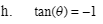Home > CCA2 > Chapter 7 > Lesson 7.2.4 > Problem7-162

7-162.
1. Find the exact value for each of the following trig expressions. For parts (g) and (h), solve the equations and assume that 0 ≤ θ ≤ 2π. Homework Help ✎

1.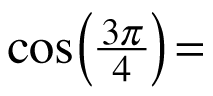2.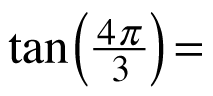3.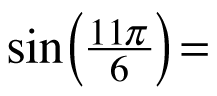4.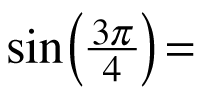5.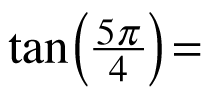6.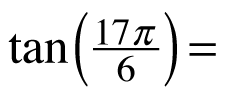7. tan(θ) = 1

8. tan(θ) = −1Refer to problem 7-123.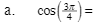$-\frac{\sqrt{2}}{2}$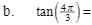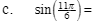$-\frac{1}{2}$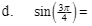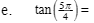1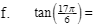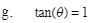$\frac{\pi}{4}$